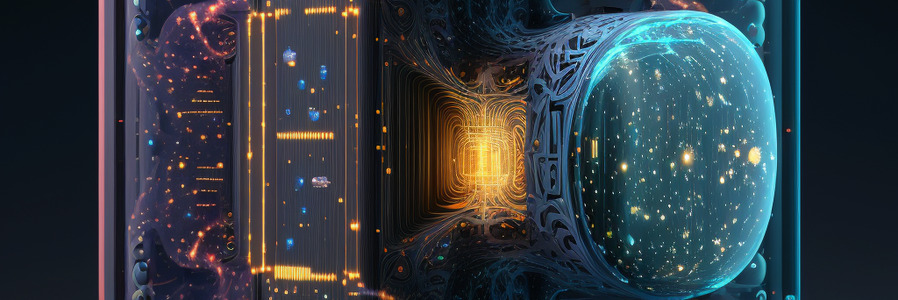# Vector Search in constant time

The advent of quantum computing has revolutionized many areas of science and technology, and one of the most intriguing developments has been its potential application to artificial neural networks (ANNs). One area where quantum computing can significantly improve performance is in vector search, a critical component of many machine learning tasks. In this article, we will discuss the concept of quantum quantization for ANN vector search, focusing on the conversion of float32 to qbit vectors and the ability to perform vector search on arbitrary-sized databases in constant time.

## Quantum Quantization and Entanglement

Quantum quantization is a novel approach that leverages the power of quantum computing to speed up the search process in ANNs. By converting traditional float32 vectors into qbit vectors, we can create quantum entanglement between the qbits. Quantum entanglement is a unique phenomenon in which the states of two or more particles become interdependent, regardless of the distance between them. This property of quantum systems can be harnessed to create highly efficient vector search algorithms.

The conversion of float32 vectors to qbit vectors can be represented by the following formula:

qbit_vector = Q( float32_vector )


where Q is the quantum quantization function that transforms the float32_vector into a quantum entangled qbit_vector.

## Vector Search in Constant Time

The primary advantage of using quantum quantization for ANN vector search is the ability to search through an arbitrary-sized database in constant time.

The key to performing vector search in constant time with quantum quantization is to use a quantum algorithm called Grover’s algorithm. Grover’s algorithm is a quantum search algorithm that finds the location of a marked item in an unsorted database in O(√N) time, where N is the size of the database. This is a significant improvement over classical algorithms, which require O(N) time to solve the same problem.

However, the is one another trick, which allows to improve Grover’s algorithm performanse dramatically. This trick is called transposition and it allows to reduce the number of Grover’s iterations from O(√N) to O(√D), where D - is a dimension of the vector space.

And since the dimension of the vector space is much smaller than the number of vectors, and usually is a constant, this trick allows to reduce the number of Grover’s iterations from O(√N) to O(√D) = O(1).

Check out our Quantum Quantization PR on GitHub.